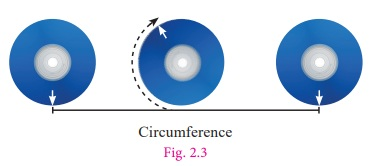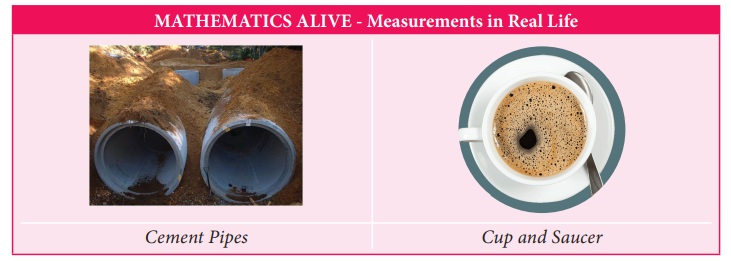Home | | Maths 7th Std | Measurements

# Measurements

Learning Objectives ● To understand the concepts of area and circumference of circle. ● To understand the area of circular and rectangular pathways.

Chapter 2

MEASUREMENTSLearning Objectives

● To understand the concepts of area and circumference of circle.

● To understand the area of circular and rectangular pathways.

Introduction

We have already studied that closed shapes such as rectangle and square having area and perimeter. Pasting of tiles on a wall, paving a parking lot with stones, fencing a field or ground etc., are some of the places where the knowledge of area and perimeter of rectangle are essential. In this chapter, we extend this concept to circles. The best example of a circle is the wheel. The invention of wheel is perhaps the greatest achievement of mankind.

The teacher shows the following pictures of wheel and asks questions as given below:Teacher : Bharath, can you tell me what is the name of the picture given in Fig 2.1?

Bharath : Yes Sir/Madam, wheel of a bicycle.

Teacher : Sathish, would you tell me what is in Fig.2.2?

Sathish : Yes Sir/Madam, it is a wheel of a car.

Teacher : Suresh, will you name the shape of both figures?

Suresh : Yes Sir/Madam, they are circular in shape.

Teacher : Yes, you are right. Now Mary, will you tell me the distance covered by the wheel when it rotates once.

Mary : I don’t know sir/madam.

Teacher : Ok, How do we measure the distance around the circle? We cannot measure the curves with the help of a ruler, as these shapes do not have straight edges. But there is a way to measure the distance around the circle. Mark a point on  its boundary. Place the wheel on the floor in such a way that the marked point coincides with the floor. Take it as the initial point. Rotate the wheel once on the floor along a straight line till the marked point again touches  the floor. The distance covered by it is the distance around the  outer edge of a circle. That is, CircumferenceSuresh :Teacher, is there any other way to find the distance?

Teacher : Yes, mark a point on its boundary and using a thread and scale we can measure  its length.

We are now going to discuss the circumference and area of the circle.

MATHEMATICS ALIVE - Measurements in Real LifeTags : Term 2 Chapter 2 | 7th Maths , 7th Maths : Term 2 Unit 2 : Measurements
Study Material, Lecturing Notes, Assignment, Reference, Wiki description explanation, brief detail
7th Maths : Term 2 Unit 2 : Measurements : Measurements | Term 2 Chapter 2 | 7th Maths